# Multiplication Games

Get ready to play some fun and interactive multiplication games! On this page we have a variety of games that kids will enjoy while building strong math skills. Whether students are learning the good old boring times-tables or even multiplying fractions or integers; we have some fun games for them on our website.

Are you tired of boring multiplication drills? Try playing these fun games instead!Multiply Numbers Ending in Zeroes

Earn points by multiplying numbers ending in zeroes to discover the hidden treasure.Multiplication Game

In this fast-paced car racing game, 2nd and 3rd grade students will practice multiplying one-digit numbers.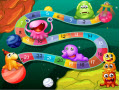Multiplication Board Game

In this fun board game, upper elementary and middle school students will practice multiplying by large numbers that are multiples of ten. The game can be played alone, in pairs, or in two teams.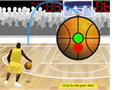Basketball Multiplication Game

Kids will have a lot of fun playing this interactive basketball game, but they will also practice multiplying one-digit whole numbers.Multiplication Concentration GamesMultiplication as Repeated Addition

In this math racing game 3rd grade students will learn to view multiplication problems as repeated addition problems.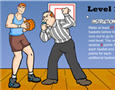Properties of Multiplication

In this math basketball game students will identify properties of multiplication and use them in mental math computations.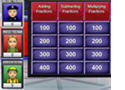Multiplication Jeopardy Game

In this jeopardy game, students will multiply one and multi-digit numbers. The game is an excellent classroom activity because it has a multi-player feature.Multiplication Basketball Game

In this game students will multiply 2-digit numbers by 1-digit numbers. The game has single- and a multi-player feature.Basketball Math - Multiplying Decimals

This is an interactive basketball game about multiplying decimals. Students can play it alone, with another classmate, or in two teams.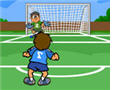Multiplying Fractions Game

In this soccer math game, students will practice multiplying fractions and writing the answer in the simplest form.